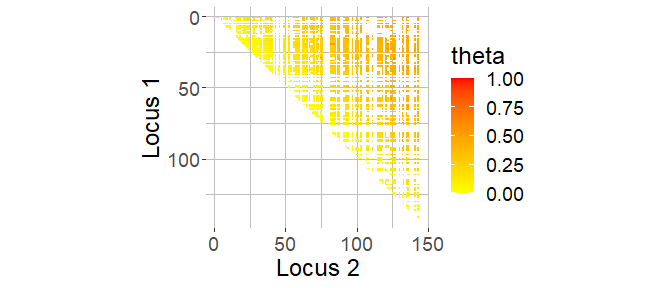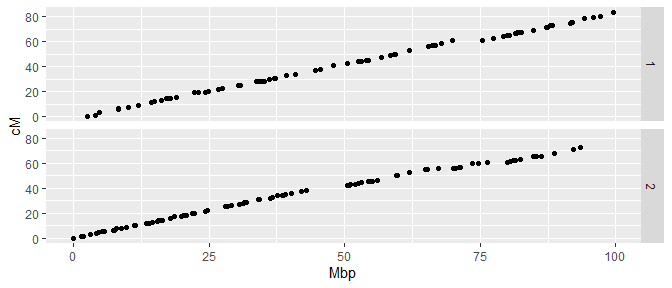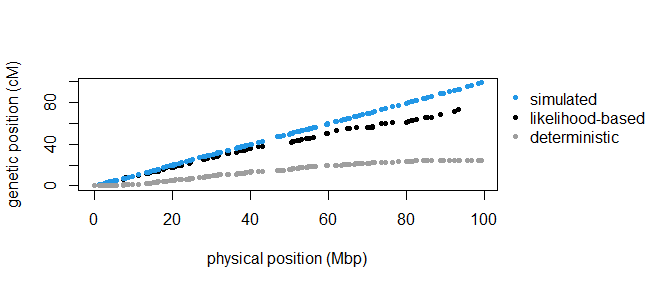# Description of the workflow for constructing a genetic map using hsrecombi

## Introduction

hsrecombi can approximate the genetic-map positions of genetic markers from SNP genotypes in half-sib families. It is referred to Hampel et al. (2018, Fron Gen) and Qanbari & Wittenburg (2020, Genet Sel Evol) for more information on the methodology. The workflow relies on paternal half-sib families but it can be adapted to maternal half-sib families with minor modifications.

In Part I, genotypic data from progeny and their common parents are simulated with the R package AlphaSimR and reshaped into the PLINK format. If genotypic data are already available in the required PLINK format, the workflow starts directly at Part II.

Required input files for Part II:

• map files for each chromosome map_chr<chr>.map
• data files for each chromosome hsphase_input_chr<chr>.raw

Note: A pipeline is available at GitHub.

library(hsrecombi)
library(AlphaSimR)
library(doParallel)
library(ggplot2)
#> Warning: package 'ggplot2' was built under R version 4.0.5
library(rlist)
Sys.time()
#>  "2022-03-25 07:46:24 CET"

### Global variables

# number of chromosomes
nchr <- 2
# Number of simulated SNPs (e.g., p = 1500 resembles an average bovine chromosome)
p <- 150
# Number of simulated progeny in each half-sib family
n <- 1000
# Directory for (simulated) data
path.dat <- "data"
dir.create(path.dat, showWarnings = FALSE)
# Directory for output
path.res <- "results"
dir.create(path.res, showWarnings = FALSE)
# Number of computing clusters allocated
nclust <- 2

For instance, executing the following workflow with $$p=1500$$ and $$n\geq 1000$$ requires about 120 Mb storage space and 10 min computing time (2.6 GHz processor). With $$p=150$$, it takes about 7 Mb and 20 sec.

## Part I: Data simulation and preparation

### 1: Simulation of genetic data with R package AlphaSimR

founderPop <- runMacs2(nInd = 1000, nChr = nchr, segSites = p)
SP <- SimParam$new(founderPop) SP$setSexes("yes_sys")
# Enable tracing location of recombination events
SP$setTrackRec(TRUE) pop <- newPop(founderPop) N <- 10 ntotal <- N * n my_pop <- selectCross(pop = pop, nFemale = 500, nMale = N, use = "rand", nCrosses = ntotal) probRec <- list() for (chr in 1:nchr) { co.pat <- matrix(0, ncol = p, nrow = ntotal) for (i in 1:ntotal) { if (nrow(SP$recHist[[1000 + i]][[chr]][]) > 1) {
# 1. line contains 1 1 by default
loci <- SP$recHist[[1000 + i]][[chr]][][-1, 2] co.pat[i, loci] <- 1 } } probRec[[chr]] <- colMeans(co.pat) } save(list = c("SP", "founderPop", "pop", "my_pop", "ntotal", "probRec"), file = "data/pop.RData") Two chromosomes, each with $$p$$ SNPs and 1 Morgan length, are simulated. The founder population consists of 1,000 animals with equal gender distribution. The follow-up generation consists of $$N$$ paternal-half sib families with equal number of progeny $$n$$. The study design with the equal number of progeny per family was preferred here for simplicity but this is not required in the subsequent steps. ### 2: Selection of sire haplotypes and progeny genotypes PAT <- my_pop@father rown <- paste(rep(unique(PAT), each = 2), c(1, 2), sep = "_") H.pat <- pullSegSiteHaplo(pop)[rown, ] X <- pullSegSiteGeno(my_pop) # Physical position of markers in Mbp map.snp <- lapply(founderPop@genMap, function(z) z * 100) Note that founderPop@genMap includes genetic positions in Morgan units but physical positions are required in the next steps. ## Part II: Main analysis cl <- makeCluster(nclust) registerDoParallel(cl) ### Steps 1-5: Calculation of pairwise recombination rates out <- foreach(chr = 1:nchr, .packages = "hsrecombi") %dopar% { # 1: Physical map map <- read.table(file.path(path.dat, paste0("map_chr", chr, ".map")), col.names = c("Chr", "Name", "locus_Mb", "locus_bp")) map$SNP <- 1:nrow(map)
locus_Mb <- map$locus_Mb # 2: Genotype matrix genomatrix <- data.table::fread(file.path(path.dat, paste0("hsphase_input_chr", chr, ".raw"))) X <- as.matrix(genomatrix[, -c(1:6)]) # 3: Assign daughters to sire IDs daughterSire <- genomatrix$PAT

# 4: Estimate sire haplotypes and format data
hap <- makehappm(unique(daughterSire), daughterSire, X)
save("hap", file = file.path(path.res, paste0("hsphase_output_chr", chr, ".Rdata")))

# Check order and dimension
io <- sapply(1:nrow(map), function(z) {
grepl(x = colnames(X)[z], pattern = map$Name[z]) }) if (sum(io) != nrow(map)) stop("ERROR in dimension") # 5: Estimate recombination rates res <- hsrecombi(hap, X, map$SNP)
final <- editraw(res, map)
save(list = c("final", "locus_Mb"), file = file.path(path.res, paste0("Results_chr", chr, ".RData")))

ifelse(nrow(final) > 0, "OK", "no result")
}
print(which(unlist(out) == "OK"))
#>  1 2

In step 4, function makehap would be sufficient but makehappm allows comparison with a deterministic approach shown later. Note that sire haplotypes can also be obtained by some other (external) software. Then sire haplotypes and sire-to-daughter information need to be reshaped with makehaplist in step 4.

### 6: Check for candidates of misplacement

# 6a: Filter SNPs with unusually large recombination rate to neighbouring (30) SNPs
excl <- foreach(chr = 1:nchr, .packages = "hsrecombi") %dopar% {
load(file.path(path.res, paste0("Results_chr", chr, ".RData")))
checkCandidates(final)
}

# 6b: Heatmap plot of recombination rates for visual verification, e.g.:
chr <- 2
load(file.path(path.res, paste0("Results_chr", chr, ".RData")))
cand <- excl[[chr]]
win <- cand + (-100:100)
win <- win[(win >= 1) & (win <= max(final$SNP2))] target <- final[(final$SNP1 %in% win) & (final$SNP2 %in% win), ] ggplot(data = target, aes(SNP2, SNP1, fill = theta)) + geom_tile() + xlab("Locus 2") + ylab("Locus 1") + coord_equal() + scale_y_continuous(trans = "reverse") + theme(panel.background = element_blank(), panel.grid.major = element_line(colour = "grey", size = 0.1), panel.grid.minor = element_line(colour = "grey")) + theme(text = element_text(size = 18)) + scale_fill_gradientn(colours = c("yellow", "red"), limits = c(0, 1 + 1e-10), na.value = "white")# -> nothing conspicious excl[] <- excl[] <- NA Orange colour in the SNP neighbourhood implies an increased recombination rate. In case of a misplaced marker or a cluster of misplaced markers, the heatmap is characterised by an orange band. ### 7: Approximation of genetic positions pos <- foreach(chr = 1:nchr, .packages = "hsrecombi") %dopar% { load(file.path(path.res, paste0("Results_chr", chr, ".RData"))) out <- geneticPosition(final, exclude = excl[[chr]]) list(pos.cM = c(out, rep(NA, length(locus_Mb) - length(out))), pos.Mb = locus_Mb) } Genetic position is reported as NA if the corresponding SNP has not been considered in the analysis (e.g., when no sire was heterozygous at the corresponding SNP). stopCluster(cl) ### 8: Plot of physical versus genetic-map positions data <- data.frame(Chr = rep(1:length(pos), times = unlist(list.select(pos, length(pos.Mb)))), Mbp = unlist(list.select(pos, pos.Mb)), cM = unlist(list.select(pos, pos.cM))) ggplot(data, aes(x = Mbp, y = cM)) + geom_point(na.rm = T) + facet_grid(Chr ~ .)### 9: Plot of genetic mapping function The genetic mapping function that fits best to the estimated data can be retrieved from a system of mapping functions (Rao et al. 1977, Human Hered); putatively misplaced markers need to be excluded. Given a parameter $$p$$, recombination rate $$\theta \in (0,0.5)$$ is mapped to Morgan units by $f(\theta; p) = \frac{1}{6}(p(2p - 1)(1 - 4p) log(1 - 2\theta) + 16p(p - 1)(2p - 1) \text{atan}(2\theta) + 2p(1 - p)(8p + 2)\text{atanh}(2\theta) + 6(1 - p)(1 - 2p)(1 - 4p)\theta)$ for (chr in 1:nchr) { load(file.path(path.res, paste0("Results_chr", chr, ".RData"))) final$theta[(final$SNP1 %in% excl[[chr]]) | (final$SNP2 %in% excl[[chr]])] <- NA
final$dist_M <- (pos[[chr]]$pos.cM[final$SNP2] - pos[[chr]]$pos.cM[final$SNP1])/100 out <- bestmapfun(theta = final$theta, dist_M = final$dist_M) plot(final$dist_M, final$theta, xlab = "genetic distance (Morgan)", ylab = "recombination rate", col = 8) x <- seq(0, 0.5, 0.01) y <- rao(out$mixing, x)
points(y, x, type = "l", lwd = 2)

### 2: Check with deterministic approach

load(file.path(path.res, paste0("hsphase_output_chr", chr, ".Rdata")))
hsphase.cM <- c(0, cumsum(hap$probRec)) * 100 The results are compared to the deterministic approach of Ferdosi et al. (2014) provided in the R package hsphase. Functions of that package are called in makehap and makehappm. ### 3: Comparative plot of physical versus genetic-map positions par(mar = c(5.1, 4.1, 4.1, 9.1), xpd = TRUE) plot(pos[[chr]]$pos.Mb, pos[[chr]]$pos.cM, xlab = "physical position (Mbp)", ylab = "genetic position (cM)", ylim = range(c(sim.cM, hsphase.cM, pos[[chr]]$pos.cM), na.rm = T), pch = 20)
points(pos[[chr]]$pos.Mb, sim.cM, pch = 20, col = 4) points(pos[[chr]]$pos.Mb, hsphase.cM, pch = 20, col = 8)
legend("topleft", inset = c(1.01, 0), legend = c("simulated", "likelihood-based", "deterministic"), pch = 20,
col = c(4, 1, 8), bty = "n")The likelihood-based approach requires a sufficiently large sample size if SNP density is high. A verification of the bias of genetic-map positions can be found in the Supplemental Material of Qanbari & Wittenburg (2020, Genet Sel Evol).

unlink(path.dat, recursive = TRUE)
unlink(path.res, recursive = TRUE)
Sys.time()
#>  "2022-03-25 07:46:42 CET"